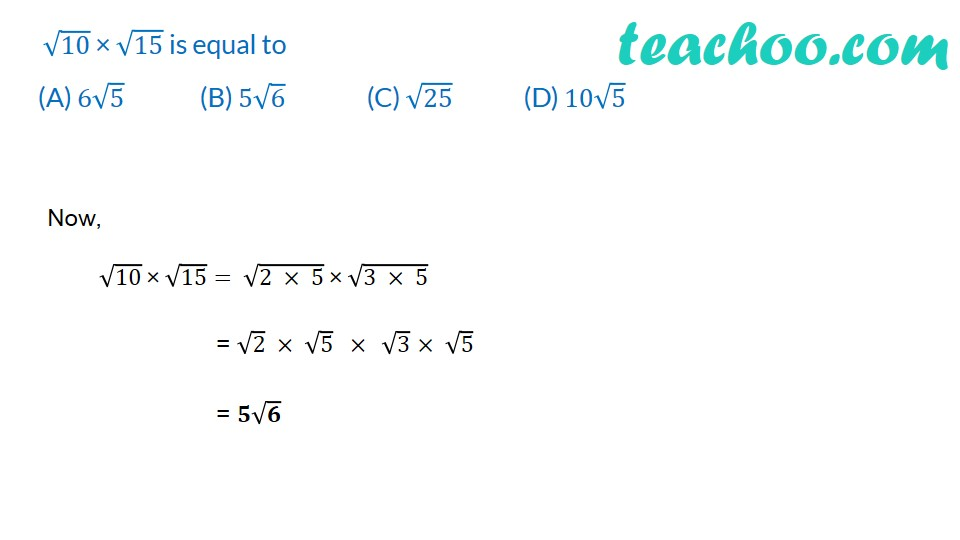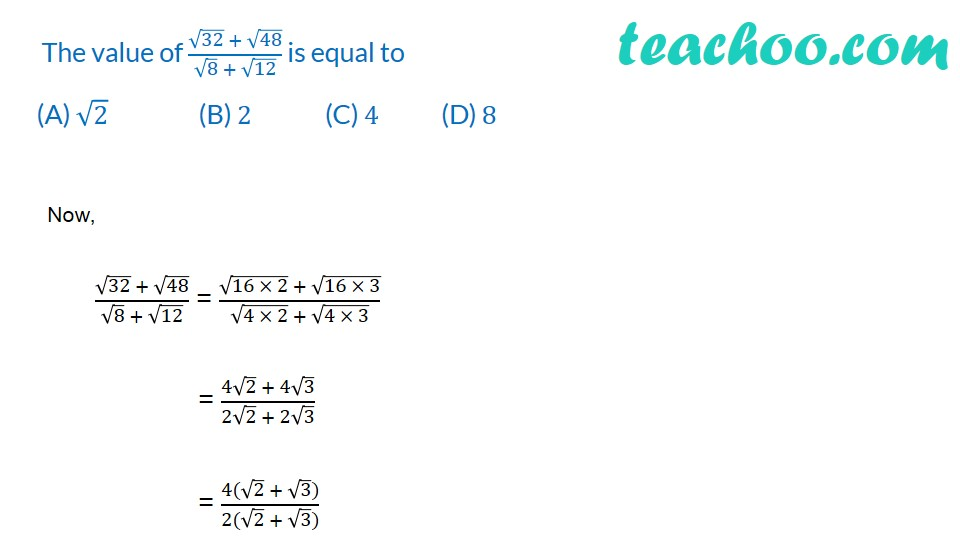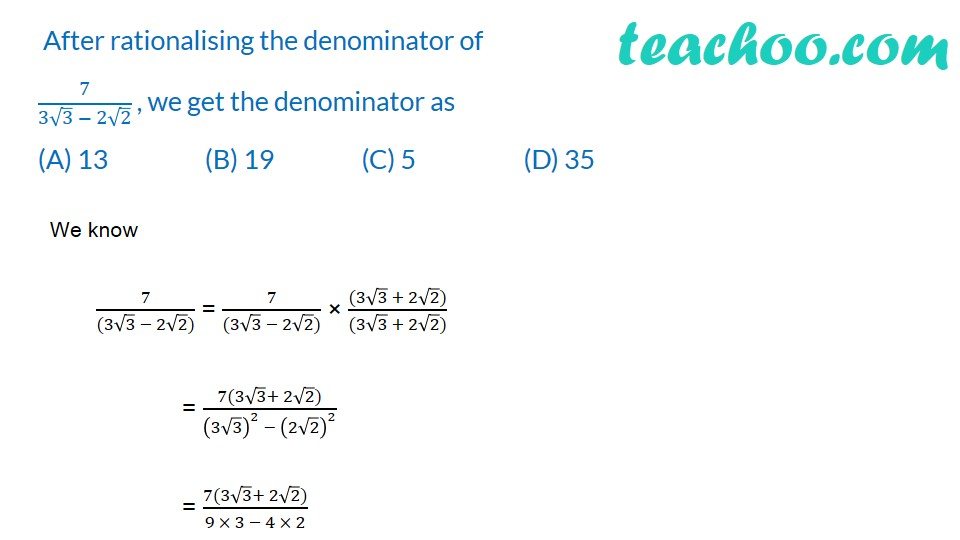Rationalising

Chapter 1 Class 9 Number Systems
Concept wiseIntroducing your new favourite teacher - Teachoo Black, at only ₹83 per month

### Transcript

﻿ √10 × √15 is equal to (A) 6√5 (B) 5√6 (C) √25 (D) 10√5 Now, ﻿ √10 × √15="﻿ " √(2 × 5) " × " √(3 × 5) = √2 × √5 " " ×" " √3 × √5 = 𝟓√𝟔 ﻿ The value of (√32 + √48)/(√8 + √12) is equal to (A) √2 (B) 2 (C) 4 (D) 8 Now, (√32 + √48)/(√8 + √12) = (√(16 × 2) + √(16 × 3))/(√(4 × 2) + √(4 × 3)) = (4√2 + 4√3)/(2√2 + 2√3) = (4(√2 + √3))/(2(√2 + √3)) ﻿ ﻿After rationalising the denominator of 7/(3√3 − 2√2) , we get the denominator as (A) 13 (B) 19 (C) 5 (D) 35 We know 7/((3√3 − 2√2)) = 7/((3√3 − 2√2)) × ((3√3 + 2√2))/((3√3 + 2√2)) = (7(3√3+ 2√2))/((3√3)^2 − (2√2)^2 ) = (7(3√3+ 2√2))/(9 × 3 − 4 × 2)